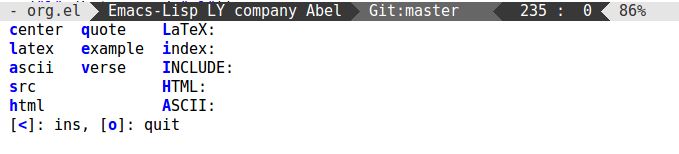# Org-mode: any keyboard shortcut for Latex code?

On p.33 of the Org-guide Carsten Dominek writes

you can use #+LATEX: and #+BEGIN_LATEX ... #+END_LATEX construct to add verbatim LATEX code

Is there a keyboard combination or default snippet that automatically types either of those constructs into the .org file in a similar fashion to `<s` followed by TAB that adds `#+BEGIN_SRC`? (see minute 3:00 in this youtube tutorial)

These shortcuts are called easy templates. There are defaults for LaTeX lines (`<L TAB`) and LaTeX blocks (`<l TAB`).

And you can add your own templates by customizing `org-structure-template-alist`.

You can make use of this hydra code to assist you in entering many Org block templates:

``````(defun hot-expand (str)
"Expand org template."
(insert str)
(org-try-structure-completion))
(defhydra hydra-org-template (:color blue :hint nil)
"
_c_enter  _q_uote    _L_aTeX:
_l_atex   _e_xample  _i_ndex:
_a_scii   _v_erse    _I_NCLUDE:
_s_rc     ^ ^        _H_TML:
_h_tml    ^ ^        _A_SCII:
"
("s" (hot-expand "<s"))
("e" (hot-expand "<e"))
("q" (hot-expand "<q"))
("v" (hot-expand "<v"))
("c" (hot-expand "<c"))
("l" (hot-expand "<l"))
("h" (hot-expand "<h"))
("a" (hot-expand "<a"))
("L" (hot-expand "<L"))
("i" (hot-expand "<i"))
("I" (hot-expand "<I"))
("H" (hot-expand "<H"))
("A" (hot-expand "<A"))
("t" (hot-expand "<t"))
("<" self-insert-command "ins")
("o" nil "quit"))
(define-key org-mode-map "<"
(defun org-self-insert-or-less ()
(interactive)
(if (looking-back "^")
(hydra-org-template/body)
(self-insert-command 1))))
``````

Here's the hint you'll see in the echo area after pressing < from beginning of line: